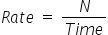Mathematics
Easy

Question

# Ron covered 560 yards in 7 seconds. __________ yards can be covered in 10 seconds

## 250400650800Hint:

## The correct answer is: 800

### Let the number of yards covered be denoted as 'N’.For first case the given values areN = 560Time = 7 seconds.To find the rate at which he is covering the land we will take the ratio of number of yards to the time.As the rate is constant, we will use this value to find the number of yards covered in 10 SecondsRearranging the equation for N, we getRate × Time = NN = Rate × Time= 80 × 10= 800So, number of yards covered in 10 seconds is 800.

For such questions, we should be careful about the units. The other way method to solve it would be to directly take the ratios and equate them. As the rate is constant, the ratio of the quantities will be constant. So we will consider the quantity we want to find as some variable, and then equate the ratios. It will be shortcut.

### Related Questions to study#### With Turito Foundation.#### Get an Expert Advice From Turito.